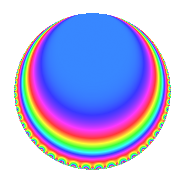Properties

 Label 3600.1.e.cLevel 3600 Weight 1 Character orbit 3600.e Analytic conductor 1.797 Analytic rank 0 Dimension 2 Projective image $$D_{6}$$ CM disc. -3 Inner twists 4

Related objects

Newspace parameters

 Level: $$N$$ = $$3600 = 2^{4} \cdot 3^{2} \cdot 5^{2}$$ Weight: $$k$$ = $$1$$ Character orbit: $$[\chi]$$ = 3600.e (of order $$2$$ and degree $$1$$)

Newform invariants

 Self dual: No Analytic conductor: $$1.79663404548$$ Analytic rank: $$0$$ Dimension: $$2$$ Coefficient field: $$\Q(\zeta_{6})$$ Coefficient ring: $$\Z[a_1, \ldots, a_{7}]$$ Coefficient ring index: $$2$$ Projective image $$D_{6}$$ Projective field Galois closure of 6.0.1440000.1

$q$-expansion

The $$q$$-expansion and trace form are shown below.

 $$f(q)$$ $$=$$ $$q$$ $$+ ( \zeta_{6} + \zeta_{6}^{2} ) q^{7}$$ $$+O(q^{10})$$ $$q$$ $$+ ( \zeta_{6} + \zeta_{6}^{2} ) q^{7}$$ $$- q^{13}$$ $$+ ( \zeta_{6} + \zeta_{6}^{2} ) q^{19}$$ $$+ ( -\zeta_{6} - \zeta_{6}^{2} ) q^{31}$$ $$-2 q^{37}$$ $$+ ( \zeta_{6} + \zeta_{6}^{2} ) q^{43}$$ $$+ ( -1 - \zeta_{6} + \zeta_{6}^{2} ) q^{49}$$ $$- q^{61}$$ $$+ ( \zeta_{6} + \zeta_{6}^{2} ) q^{67}$$ $$+ 2 q^{73}$$ $$+ ( -\zeta_{6} - \zeta_{6}^{2} ) q^{91}$$ $$- q^{97}$$ $$+O(q^{100})$$ $$\operatorname{Tr}(f)(q)$$ $$=$$ $$2q$$ $$\mathstrut +\mathstrut O(q^{10})$$ $$2q$$ $$\mathstrut -\mathstrut 2q^{13}$$ $$\mathstrut -\mathstrut 4q^{37}$$ $$\mathstrut -\mathstrut 4q^{49}$$ $$\mathstrut -\mathstrut 2q^{61}$$ $$\mathstrut +\mathstrut 4q^{73}$$ $$\mathstrut -\mathstrut 2q^{97}$$ $$\mathstrut +\mathstrut O(q^{100})$$

Character Values

We give the values of $$\chi$$ on generators for $$\left(\mathbb{Z}/3600\mathbb{Z}\right)^\times$$.

 $$n$$ $$577$$ $$901$$ $$2801$$ $$3151$$ $$\chi(n)$$ $$1$$ $$1$$ $$1$$ $$-1$$

Embeddings

For each embedding $$\iota_m$$ of the coefficient field, the values $$\iota_m(a_n)$$ are shown below.

For more information on an embedded modular form you can click on its label.

Label $$\iota_m(\nu)$$ $$a_{2}$$ $$a_{3}$$ $$a_{4}$$ $$a_{5}$$ $$a_{6}$$ $$a_{7}$$ $$a_{8}$$ $$a_{9}$$ $$a_{10}$$
3151.1
 0.5 − 0.866025i 0.5 + 0.866025i
0 0 0 0 0 1.73205i 0 0 0
3151.2 0 0 0 0 0 1.73205i 0 0 0
 $$n$$: e.g. 2-40 or 990-1000 Significant digits: Format: Complex embeddings Normalized embeddings Satake parameters Satake angles

Inner twists

Char. orbit Parity Mult. Self Twist Proved
1.a Even 1 trivial yes
3.b Odd 1 CM by $$\Q(\sqrt{-3})$$ yes
4.b Odd 1 yes
12.b Even 1 yes

Hecke kernels

This newform can be constructed as the intersection of the kernels of the following linear operators acting on $$S_{1}^{\mathrm{new}}(3600, [\chi])$$:

 $$T_{7}^{2}$$ $$\mathstrut +\mathstrut 3$$ $$T_{13}$$ $$\mathstrut +\mathstrut 1$$